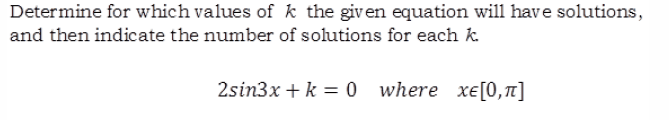# Solve for trig equation with 2 variables

## Homework Statement## The Attempt at a Solution

I can solve the first part of the question. -2 ≤ k ≤ 2 because -1 ≤ sin(x) ≤ 1. How do I solve the second part of the question? Thanks.

Forget k for a moment, and think about what values 2 sin(3x) can assume. For each such value v, you have 2 sin(3x) = v, or 2 sin(3x) + (-v) = 0. So your k values are the same as the values of -v.

Forget k for a moment, and think about what values 2 sin(3x) can assume. For each such value v, you have 2 sin(3x) = v, or 2 sin(3x) + (-v) = 0. So your k values are the same as the values of -v.

It may help to think about the graph of this function. It may even help to graph it. The 2 will increase the amplitude of the function and the 3 will scale the function. More specifically, it will "shrink" the function in the x direction (but it will not affect affect the amplitude). So from 0 to pi, the function will go completely up and down once and then be halfway done with a cycle. If you draw a horizontal line through this equation, how many solutions are there? (Hint: it depends on where the line is).

http://www4c.wolframalpha.com/Calculate/MSP/MSP4251a45574034148cah00001164cg2h50a94c78?MSPStoreType=image/gif&s=5&w=300&h=183&cdf=RangeControl [Broken]

So if k:
is = -2, there will be 2 zeros
is between (-2,0] there will be 4 zeros
is between (0,2), there will be 2 zeros
is = 2, there will be 1 zero

Is that correct? Thanks.

Last edited by a moderator:
http://www4c.wolframalpha.com/Calculate/MSP/MSP4251a45574034148cah00001164cg2h50a94c78?MSPStoreType=image/gif&s=5&w=300&h=183&cdf=RangeControl [Broken]

So if k:
is = -2, there will be 2 zeros
is between (-2,0] there will be 4 zeros
is between (0,2), there will be 2 zeros
is = 2, there will be 1 zero

Is that correct? Thanks.

That looks correct to me, but you should check your result by looking at a graph of the function, perhaps with k=0 if you haven't already done so.

One other small matter, which you probably take for granted, but you should still mention if this is a homework or exam problem: what happens when k > 2 or k < -2?

Last edited by a moderator:
http://www4c.wolframalpha.com/Calculate/MSP/MSP4251a45574034148cah00001164cg2h50a94c78?MSPStoreType=image/gif&s=5&w=300&h=183&cdf=RangeControl [Broken]

So if k:
is = -2, there will be 2 zeros
is between (-2,0] there will be 4 zeros
is between (0,2), there will be 2 zeros
is = 2, there will be 1 zero

Is that correct? Thanks.

Very close. However, the only time that there are 4 solutions is when k=0. For k (-2,0), there are 3 solutions

Last edited by a moderator:
Very close. However, the only time that there are 4 solutions is when k=0. For k (-2,0), there are 3 solutions

There are never only 3 solutions. For -2 < k < 0 just consider k = -1 and test x = π/18, 5π/18, 13π/18, 17π/18.

Oops! Then what he said was correct

That looks correct to me, but you should check your result by looking at a graph of the function, perhaps with k=0 if you haven't already done so.

One other small matter, which you probably take for granted, but you should still mention if this is a homework or exam problem: what happens when k > 2 or k < -2?

You're right, I forgot to mention that. When k > 2 or k < 2, there are no solutions (because the amplitude is 2).

Additionally, could I approach this problem algebraically (without referring to a graph)?

Thanks.

Never mind. Thank you Michael & piercebeatz.